Home

# Early-time periodic injection and extraction in an inclined confined aquifer

## Abstract

We consider the periodic injection and extraction of fluid from a horizontal line well in an inclined saturated aquifer of finite thickness,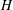$H$ , as part of an aquifer thermal energy storage system. We focus on the case in which the injected fluid is dense relative to the original fluid in the aquifer and hence tends to run off downslope, so that during the extraction cycle a mixture of original aquifer fluid and injected fluid is produced. We study the controls on the composition of the extracted fluid, distinguishing between the cases where there is an open boundary downslope or upslope of the source, with the other boundary being sealed. We find that initially the fraction of original aquifer fluid recovered during an extracted cycle decreases from cycle to cycle, and at long times this fraction asymptotes to a value,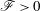$\mathscr{F}>0$ , that depends on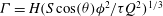${\it\Gamma}=H(S\cos ({\it\theta}){\it\phi}^{2}/{\it\tau}Q^{2})^{1/3}$ and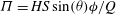${\it\Pi}=HS\sin ({\it\theta}){\it\phi}/Q$ , where${\it\tau}$ is the time period of injection,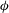${\it\phi}$ is the porosity of the aquifer,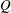$Q$ is the injection rate,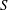$S$ is the speed of the buoyancy driven flow and${\it\theta}$ is the angle of inclination to the horizontal. At long times the flow asymptotes to a quasi-steady exchange flow solution that develops in an inclined saturated porous layer of thickness$H$ in contact with a stratified fluid reservoir, filled to thickness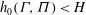$h_{0}({\it\Gamma},{\it\Pi})<H$ with relatively dense fluid. This enables us to calculate the average composition of the extracted fluid, which is of concern if the original aquifer fluid contains contaminants. Since the produced aquifer fluid is driven by this quasi-steady exchange flow, we show that contaminants downslope of the well will be produced after a finite time, whereas contaminants upslope of the well typically remain in the aquifer, irrespective of the far-field boundary conditions. We successfully test the models with a series of analogue experiments of both the injection extraction flow and the exchange flow using a Hele-Shaw cell.

## References

Hide All
MathJax
MathJax is a JavaScript display engine for mathematics. For more information see http://www.mathjax.org.

# Early-time periodic injection and extraction in an inclined confined aquifer

## Metrics

### Full text viewsFull text views reflects the number of PDF downloads, PDFs sent to Google Drive, Dropbox and Kindle and HTML full text views.

Total number of HTML views: 0
Total number of PDF views: 0 *Loading metrics...

### Abstract viewsAbstract views reflect the number of visits to the article landing page.

Total abstract views: 0 *Loading metrics...

* Views captured on Cambridge Core between <date>. This data will be updated every 24 hours.

Usage data cannot currently be displayed.